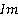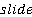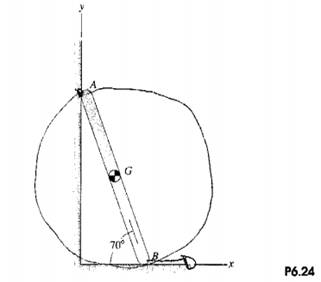### Create an Account

Home / Questions / Points A and B of the bar on the plane surfaces The velocity of point B is 2 ms a What...

# Points A and B of the bar on the plane surfaces The velocity of point B is 2 ms a What is the angular velocity vector of the bar b What is the velocity of point

Points A and B of thebaron the plane surfaces. The velocity of point B is 2m/s.

(a) What is the angular velocity vector of the bar?

(b) What is the velocity of point A?Jun 16 2020 View more View LessSubscribe To Get Solution